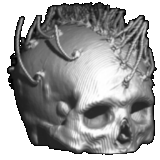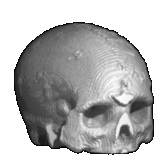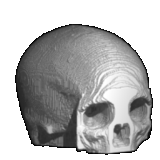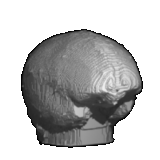A set of phantom data is available for other researchers to use in evaluating and comparing different forward and inverse methods for EEG and MEG. This directory contains all the EEG and MEG data files related to our human skull phantom studies. We have stored the data in MATLAB formats to facilitate cross platform compatibility. The phantom design and data collection procedures are described in detail in the paper: R. Leahy, J.C. Mosher, M. Spencer, M.X. Huang, J. Lewine, "A study of dipole localization accuracy for MEG and EEG using a human skull phantom" to appear: Electroencephalography and Clinical Neurophysiology. See the publications page to view or download a PDF or postscript version of this paper.

(You must right-click on a filename and then choose "Save Link As")Here is some detailed information about the data files used in our human skull analyses. Most of the data files are in the *.mat format, so one can load them into MATLAB independent of which operating system he/she is using.

The following list contains the names of the files and the description of the individual variables.

1) surface_data.mat: raw surface data files in CT coordinate system.

• scalp (210983 x 3) x,y,z points for scalp.
• skull (186406 x 3) x,y,z points for skull.
• brain (125359 x 3) x,y,z points for brain.

2) mesh_data.mat: tessellated surface data in both CT and patient coordinate system (PCS). The meshes in PCS system are in meters.

• mesh_scalp_ct (1148 x 3) x,y,z of triangular mesh nodes for scalp in CT system.
• mesh_skull_ct (1148 x 3) x,y,z of triangular mesh nodes for skull in CT system.
• mesh_brain_ct (1148 x 3) x,y,z of triangular mesh nodes for brain in CT system.
• mesh_scalp (1148 x 3) x,y,z of triangular mesh nodes for scalp in PCS system.
• mesh_skull (1148 x 3) x,y,z of triangular mesh nodes for skull in PCS system.
• mesh_brain (1148 x 3) x,y,z of triangular mesh nodes for brain in PCS system.

3) CT_PCS_trans.mat: this file contains the information that one needs to transform a point (or points) from CT coordinate to PCS. We used the electrodes identified from CT slices and those measured in patient coordinate system to form the transformation. Since dipole #59 is outside the FOV of the CT scans it was not included in the calculation. If A_CT is a 3 by M location matrix in CT system, to transform A_CT into PCS to form A_PCS (also M by 3), one can use:
>> A_PCS = scale_ct2pcs*Q_ct2pcs' * (A_CT-CT_cen*ones(1,M)) + PCS_cen*ones(1,M);

One the other hand, to transform from PCS into CT system, one can use:
>> A_CT = scale_pcs2ct*Q_pcs2ct' * (A_PCS-PCS_cen*ones(1,M)) + CT_cen*ones(1,M);

• eeg_ct_all (64 x 4) the 1st column is the EEG electrode index. Dipole #59 is excluded. The rest 3 columns are the coordinates in the CT system.
• eeg_pcs (65 x 3) x,y,z coordinates of the 65 EEG electrodes measured in PCS in mm.
• eeg_ct2pcs (65 x 3) x,y,z coordinates of the 65 EEG electrodes identified from CT and transformed to PCS in mm.
• CT_cen, PCS_cen, Q_ct2pcs, Q_pcs2ct, scale_ct2pcs, scale_pcs2ct are variables used in the transformations.

4) forward_BEM.mat: forward calculation of MEG and EEG for a dipole grid using BEM model. For the MEG, 4 out of 122 channels were bad channels, hence, the gain was calculated for the 118 good channels.

• gain_grid_BEM_eeg (64 x 3444) gain matrix for EEG BEM.
• gain_grid_BEM_meg (118 x 3444) gain matrix for MEG BEM.
• grid_dip_BEM (1148 x 3) x,y,z of dipole grid for BEM in PCS.

5) forward_2sph.mat: forward calculation of MEG and EEG for a dipole grid using 2-locally fitted spherical model.

• center1 (1 x 1) best fitting center for left-center area.
• center2 (1 x 1) best fitting center for left-occipital area.
• conduct (1 x 3) conductivities for scalp, skull, brain.
• radius_sph1 (1 x 3) radius of 3-concentric spheres for left- center area.
• radius_sph2 (1 x 3) the radius of 3-concentric spheres for left- occipital area.
• dip_cen_id (32 x 1) indicate which spherical center for which dipole. For example, "dip_cen_id(1) = 1" means "center1" should be used for dipole #1.
• eeg_pcs_center1 (65 x 3) the 65 EEG electrodes mapped to the spherical scalp with "center1" as the center. The 65th electrode is the reference electrode.
• eeg_pcs_center2 (65 x 3) the 65 EEG electrodes mapped to the spherical scalp with "center2" as the center. The 65th electrode is the reference electrode.
• grid_dip_centered (2016 x 3) x,y,z of dipole grid for 2-locally fitted spherical model.
• gain_grid_2sph_eeg1 (64 x 6048) EEG gain matrix using "center1"
• gain_grid_2sph_eeg2 (64 x 6048) EEG gain matrix using "center2"
• gain_grid_2sph_meg1 (118 x 6048) MEG gain matrix using "center1"
• gain_grid_2sph_meg2 (118 x 6048) MEG gain matrix using "center2"

6) true_loc_ori.mat: true locations and orientations of dipoles.

• dip_loc_ct_true (32 x 3) true dipole locations in CT system.
• dip_ori_ct_true (32 x 3) true dipole orientations in CT system.
• dip_loc_pcs_true (32 x 3) true dipole locations in PCS system.
• dip_ori_pcs_true (32 x 3) true dipole orientations in PCS.

7) phantom_eeg_meg.mat: contains the EEG and MEG data for the human skull phantom.

• eeg_pcs (65 x 3) EEG electrode locations in x,y,z. The first 64 rows are for the 64 measurement electrodes. The last one is the reference electrode.
• eeg_ct2pcs (65 x 3) EEG electrode locations in x,y,z. The first 64 rows are for the 64 electrodes identified from CT and transformed into PCS. The last one is the reference electrode.
• R (118 x 6) MEG sensor locations in x,y,z. The original MEG data were collected using Neuromag 122 with 122 planar gradiometers. Four bad channels were deleted. Each of the remaining 188 channels contains two pick-up coils. So, the first 3 columns are for the first coils, and the next 3 columns for the second coils.
• O (118 x 6) corresponding MEG sensor orientations.
• EEG_latency1, EEG_latency2, ..., EEG_latency32 (1 x 176): the EEG time latencies for dipole 1, 2, ..., 32.
• EEGwf1, EEGwf2, ..., EEGwf32 (64 x 176): EEG waveforms for dipole 1, 2,...,32. Each row is for one EEG channel.
• MEG_latency1, MEG_latency2, ..., MEG_latency32 (1 x 351): the MEG time latencies for dipole 1, 2, ..., 32.
• MEGwf1, MEGwf2, ..., MEGwf32 (118 x3516): MEG waveforms for dipole 1, 2,...,32. Each row is for one MEG channel.

8) jack_256x256x325.bin: The raw CT data, isotropically resampled CT scans.

• The file contains a stack of 325 coronal sections of the phantom with a maximum gray level of 255 and resolution of 256x256. The voxel size is 0.58x0.58x0.58 (mm^3).
The file is in binary format and each voxel is stored as a single byte. The following pseudo-code can be used to load and process the image slice by slice:
File_Handle = FOPEN('jack_256x256x325.chr','BINARY_MODE')
FOR K = 1 TO 325
FSEEK(File_Handle, k*256*256, SEEK_FIRST) Image = FREAD(File_Handle, 256*256, 'UCHAR')
.... process(Image) ....
NEXT K

9) jack_256x256x325.bin.gz: Compressed raw CT data, isotropically resampled CT scans.

• This file may reduce the download time by half but you must use the UNIX program "gunzip" to restore the file.